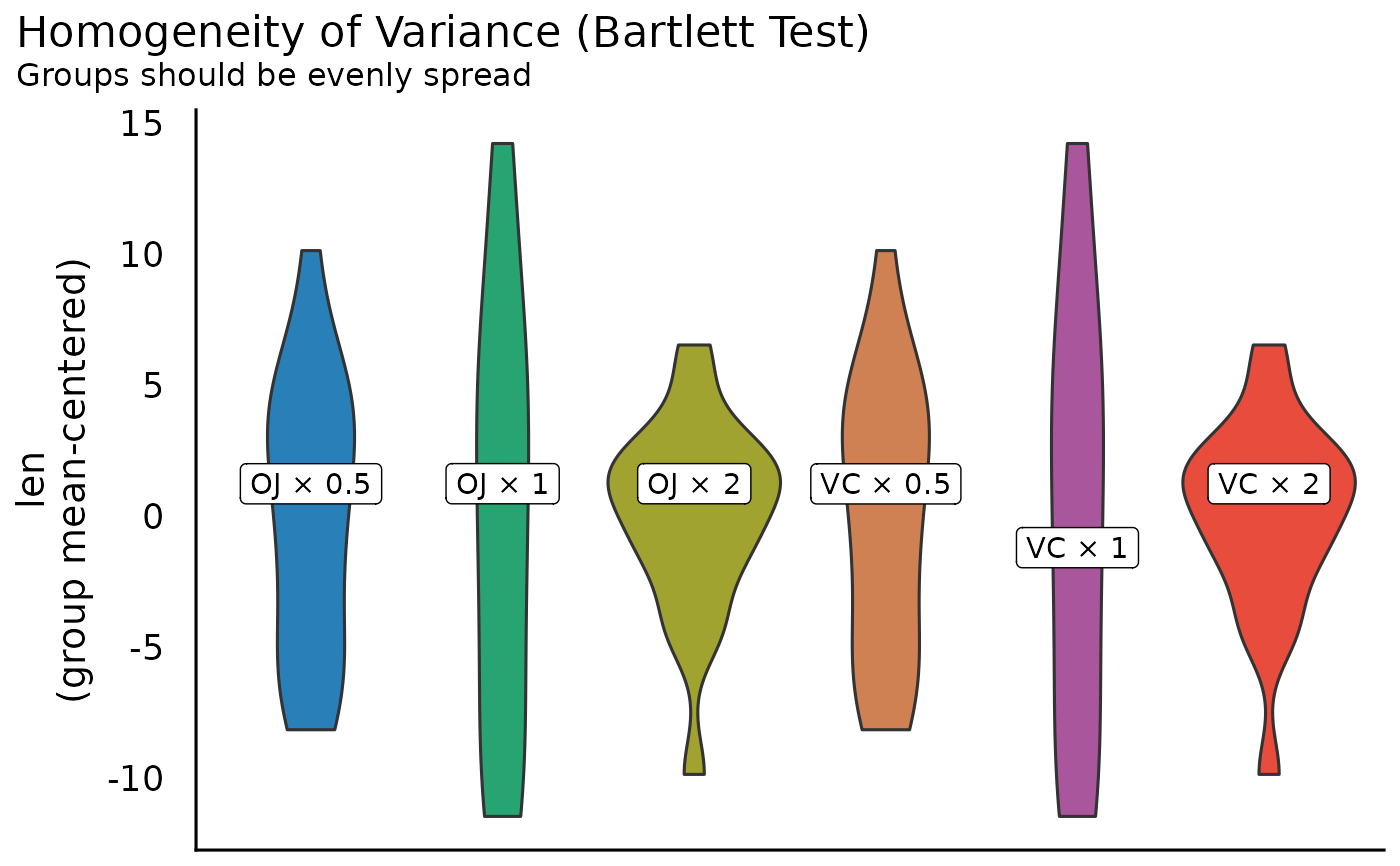Check model for homogeneity of variances between groups described by independent variables in a model.

## Usage

check_homogeneity(x, method = c("bartlett", "fligner", "levene", "auto"), ...)

# S3 method for afex_aov
check_homogeneity(x, method = "levene", ...)

## Arguments

x

A linear model or an ANOVA object.

method

Name of the method (underlying test) that should be performed to check the homogeneity of variances. May either be "levene" for Levene's Test for Homogeneity of Variance, "bartlett" for the Bartlett test (assuming normal distributed samples or groups), "fligner" for the Fligner-Killeen test (rank-based, non-parametric test), or "auto". In the latter case, Bartlett test is used if the model response is normal distributed, else Fligner-Killeen test is used.

...

Arguments passed down to car::leveneTest().

## Value

Invisibly returns the p-value of the test statistics. A p-value < 0.05 indicates a significant difference in the variance between the groups.

## Note

There is also a plot()-method implemented in the see-package.

Other functions to check model assumptions and and assess model quality: check_autocorrelation(), check_collinearity(), check_convergence(), check_heteroscedasticity(), check_model(), check_outliers(), check_overdispersion(), check_predictions(), check_singularity(), check_zeroinflation()

## Examples

model <<- lm(len ~ supp + dose, data = ToothGrowth)
check_homogeneity(model)
#> OK: There is not clear evidence for different variances across groups (Bartlett Test, p = 0.226).
#>

# plot results
if (require("see")) {
result <- check_homogeneity(model)
plot(result)
}